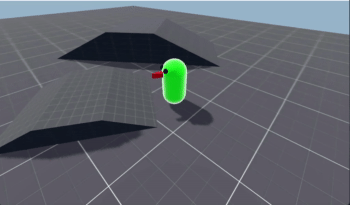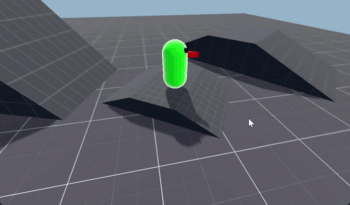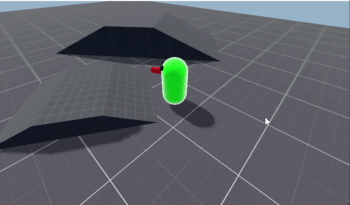# KinematicBody: Stopping on Slopes

## Problem

Your `KinematicBody` slides down slopes.

## Solution

We’ve started with a no-frills `KinematicBody`, using `move_and_slide()`, using the script below:

``````extends KinematicBody

export var gravity = -10.0
export var speed = 5.0
export var rot_speed = 4.0
export var jump_speed = 5.0

var velocity = Vector3.ZERO
var jumping = false

func get_input(delta):
var input = Vector3.ZERO
if Input.is_action_pressed("forward"):
input += -transform.basis.z * speed
if Input.is_action_pressed("back"):
input += transform.basis.z * speed
if Input.is_action_pressed("right"):
rotate_y(-rot_speed * delta)
if Input.is_action_pressed("left"):
rotate_y(rot_speed * delta)
velocity.x = input.x
velocity.z = input.z

func _physics_process(delta):
get_input(delta)
velocity.y += gravity * delta

velocity = move_and_slide(velocity, Vector3.UP)

if jumping and is_on_floor():
jumping = false

if Input.is_action_just_pressed("jump"):
if is_on_floor():
jumping = true
velocity.y = jump_speed
``````

We see the problem if we stop moving on a slope:This is `move_and_slide()` doing what it’s supposed to do.

The downward velocity caused by gravity is being slid along the surface.

Checking the `move_and_slide()` documentation, we see there’s a parameter called `stop_on_slope`, which defaults to `false`:

If `stop_on_slope` is true, body will not slide on slopes when you include gravity in linear_velocity and the body is standing still.

So we can change our movement to this instead:

``````velocity = move_and_slide(velocity, Vector3.UP, true)
``````

Now we stop sliding down slopes!But there is still a problem, which is easier to see if you use a low value for `gravity`:When we come to a stop, we have a little bit of upward momentum, which causes the small “hop”. We can solve this by switching to the `move_and_slide_with_snap()` method.

In order to ensure we can still jump, we also need to disable snapping during a jump, or we’ll remain “snapped” to the ground:

``````    var snap = Vector3.DOWN if not jumping else Vector3.ZERO
velocity = move_and_slide_with_snap(velocity, snap, Vector3.UP, true)
``````

Now the “hop” is gone, and everything works as expected.

Finally, you may notice that on very steep slopes, you still have a problem:This is because the default value of the `floor_max_angle` parameter is 45°, and the slope shown is greater. Any angle above this value does not count as a floor. Increasing the value makes this slope behave like the others:

``````velocity = move_and_slide_with_snap(velocity, snap, Vector3.UP,